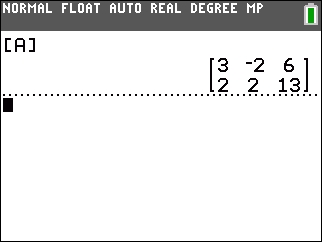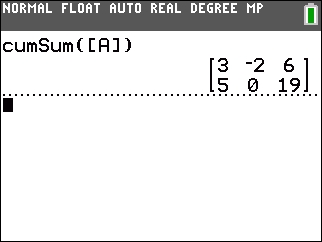# Knowledge Base

## Solution 34491: Calculating the Cumulative Sum of a Matrix on the on the TI-84 Plus C Silver Edition Graphing Calculator.

### How do I find the cumulative sum of a matrix on a TI-84 Plus C Silver Edition graphing calculator?

To find the cumulative sum of a matrix, input the matrix, exit the matrix editor, and then paste the cumsum() function on the home screen with the name of the matrix. The example below will demonstrate this procedure.

Example: Find the cumsum() of matrix [3, -2, 6; 2, 2, 13]

Solution:

To enter the matrix:
• Press [2nd] [MATRIX] or [MATRX]
• Scroll to Edit
• Press  to access matrix A
• Input the dimensions  [ENTER]  [ENTER]
• Input the matrix entries, pressing enter after each entry as shown below
• Exit the matrix editor by selecting [2nd] [QUIT]To find the cumulative sum using the cumsum( function:
• Press [2nd] [MATRIX]  or [MATRX]
• Scroll to MATH
• Press  [2nd] [MATRIX]  [)] [ENTER]Please see the TI-84 Plus C Silver Edition guidebook for additional information.